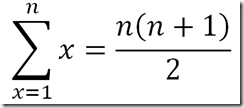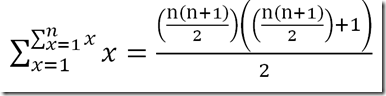# .ToString(theory);

My travels in the .Net underworld

Home  |   Contact  |   Syndication|   Login19 Posts | 0 Stories | 0 Trackbacks

### Image Galleries

I gotta admit, I am kind of surprised that I didn’t realize I could do this sooner.  I recently had a problem which required a recursive function call to come up with the answer.  After some time messing around with a recursive method, and creating an API that I was not happy with, I was able to create an API that I enjoy, and seems intuitive.

# Introduction

To bring it to a simple example, consider the summation to n:A mathematically identical formula is:In a .NET function, this can be represented by a function:

`Func<int, int> summation = x => x*(x+1)/2`

Calling summation with an input integer will yield the summation to that number:

```var sum10 = summation(4);
//sum10 would be equal to 10```

But what if I wanted to get a second level summation…  First sum to n, and then use that argument as the input to the same function, to find the second level summation:So as an easy example, calculate the summation to 3, which yields 6.  Then calculate the summation to 6 which yields 21.

Represented as a mathematical formula -So what if I wanted to represent this as .NET functions?  I can always do:

```//using the summation formula from above
var sum3 = summation(3); //sets sum3 to 6
var sum3_2 = summation(sum3); //sets sum3 to 21```

I could always create a while loop to perform the calculations too:
```Func<int, int> summation = x => x*(x+1)/2;

//for the interests of a smaller example, using shorthand

int sumResultTo = 3;

int level = 2;

while(level-- > 0)
sumResultTo = summation(sumResultTo);

//sumResultTo is equal to 21 now.```

Or express it as a for-loop, method calls, etc…  I really didn’t like any of the options that I tried.  Then it dawned on me – since I was using a Func<T, T> anyways, why not use the Func’s output from one call as the input to another directly.

# Some Code

So, I decided that I wanted a recursion class.  Something that would be generic and reusable in case I ever wanted to do something like this again. It is limited to only the Func<T1, T2> overload of Func, and T1 must be the same as T2.

The first thing in this class is a private field for the function:

`private readonly Func<T, T> _functionToRecurse;`

So, since I want the function to be unchangeable, I have defined it as readonly.  Therefore my constructor looks like:

```public Recursion(Func<T, T> functionToRecurse)
{
if (functionToRecurse == null)
{
throw new ArgumentNullException("functionToRecurse", "The function to recurse can not be null");
}
_functionToRecurse = functionToRecurse;
}```

Simple enough. Next, I want to be able to get the result of a function dependent on how many levels of recursion:

```private Func<T, T> GetXLevel(int level)
{
if (level < 1)
throw new ArgumentOutOfRangeException("level", level, "The level of recursion must be greater than 0");

```
```    if (level == 1)
return _functionToRecurse;
return _GetXLevel(level - 1, _functionToRecurse);
}```

So, if you pass in 1 for the level, you get just the Func<T,T> back.  If you say that you want to go deeper down the rabbit hole, it calls a method which accepts the level it is at, and the function which it needs to use to recurse further:

```private Func<T, T> _GetXLevel(int level, Func<T, T> prevFunc)
{
if (level == 1)
return y => prevFunc(_functionToRecurse(y));
return _GetXLevel(level - 1, y => prevFunc(_functionToRecurse(y)));
}```

That is really all that is needed for this class. If I exposed the GetXLevel function publicly, I could use that to get the function for a level, and pass in the argument..  But I wanted something cleaner.  So, I used the ‘this’ array operator for the class:

```public Func<T,T> this[int level]
{
get
{
if (level < 1)
{
throw new ArgumentOutOfRangeException("level", level, "The level of recursion must be greater than 0");
}
return this.GetXLevel(level);
}
}```

So, using the same example above of finding the second recursion of the summation of 3:

```var summator = new Recursion<int>(x => (x * (x + 1)) / 2);
var sum_3_level2 = summator(3); //yields 21```

You can even just store the delegate to the second level summation, and use it multiple times:

```var summator = new Recursion<int>(x => (x * (x + 1)) / 2);
var sum_level2 = summator;
var sum_3_level2 = sum_level2(3); //yields 21
var sum_4_level2 = sum_level2(4); //yields 55
var sum_5_level2 = sum_level2(5); //yields 120```

# Full Code

Don’t think I was just going to hold off on the full file together and make you do the hard work…  Copy this into a new class file:

```public class Recursion<T>
{

public Recursion(Func<T, T> functionToRecurse)
{
if (functionToRecurse == null)
{
throw new ArgumentNullException("functionToRecurse", "The function to recurse can not be null");
}
_functionToRecurse = functionToRecurse;
}

public Func<T,T> this[int level]
{
get
{
if (level < 1)
{
throw new ArgumentOutOfRangeException("level", level, "The level of recursion must be greater than 0");
}
return this.GetXLevel(level);
}
}

private Func<T, T> GetXLevel(int level)
{
if (level < 1)
{
throw new ArgumentOutOfRangeException("level", level, "The level of recursion must be greater than 0");
}
if (level == 1)
return _functionToRecurse;
return _GetXLevel(level - 1, _functionToRecurse);
}

private Func<T, T> _GetXLevel(int level, Func<T, T> prevFunc)
{
if (level == 1)
return y => prevFunc(_functionToRecurse(y));
return _GetXLevel(level - 1, y => prevFunc(_functionToRecurse(y)));
}
}```

# Conclusion

The great thing about this class, is that it can be used with any function with same input/output parameters.  I strived to find an implementation that I found clean and useful, and I finally settled on this.  If you have feedback – good or bad, I would love to hear it!

posted on Thursday, June 21, 2012 8:23 PM